# Graphs for Nonnormal Capability Sixpack

Find definitions and interpretation guidance for every graph that is provided with the nonnormal capability sixpack.

## Xbar Chart

The Xbar chart plots the average of the measurements within each subgroup. The center line is the average of all subgroup averages. The control limits, which are set at a distance of 3 standard deviations above and below the center line, show the amount of variation that is expected in the subgroup averages.

Minitab displays an Xbar chart when your subgroup size is greater than 1.

### Interpretation

Use an Xbar chart to monitor the mean of a process and determine whether it is stable enough to perform capability analysis.

Points located beyond the red control limits indicate that the process may not be stable and that the results of a capability analysis may not be reliable. You should identify the cause of out-of-control points and eliminate special-cause variation before you analyze process capability.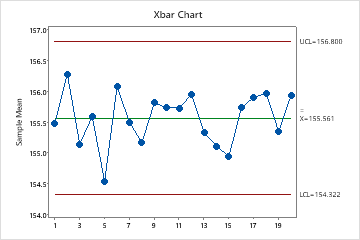In these results, the points vary randomly around the center line and are within the control limits. No trends or patterns are present. The process center is stable enough to perform capability analysis.

## I Chart

The I chart plots individual observations. The center line is an estimate of the process average. The control limits, which are set at a distance of 3 standard deviations above and below the center line, show the amount of variation that is expected in the individual sample values.

Minitab displays an I chart when your subgroup size is 1.

### Interpretation

Use an I chart to assess whether the individual measurements are in control and determine whether the process is stable enough to perform capability analysis.

Points located beyond the red control limits indicate that the process may not be stable and that the results of a capability analysis may not be reliable. You should identify the cause of out-of-control points and eliminate special-cause variation before you analyze process capability.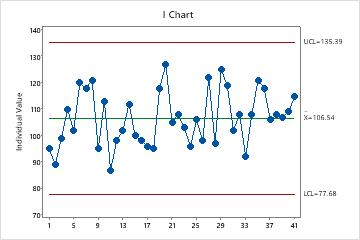In this chart, the points vary randomly around the center line and are within the control limits. No trends or patterns are present. The process center is stable enough to perform capability analysis.

## R Chart

The R chart plots the subgroup ranges. If the subgroup size is constant, then the center line is the average of all subgroup ranges. If the subgroup sizes differ, then the value of the center line depends on the subgroup size, because larger subgroups tend to have larger ranges. The control limits, which are set at a distance of 3 standard deviations above and below the center line, show the amount of variation that is expected in the subgroup ranges.

Minitab displays an R chart if your subgroup size is greater than 2 but less than 9.

### Interpretation

Use an R chart to monitor the variation of a process and determine whether it is stable enough to perform capability analysis. The R chart indicates whether the subgroup-to-subgroup variation is in control.

Points located beyond the red control limits indicate that the process may not be stable and that the results of a capability analysis may not be reliable. You should identify the cause of out-of-control points and eliminate special-cause variation before you analyze process capability.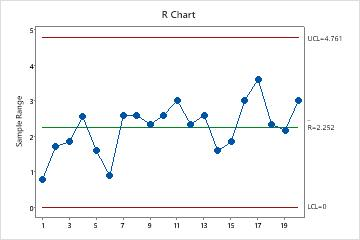In these results, the points vary randomly around the center line and are within the control limits. No trends or patterns are present. The process variation is stable enough to perform capability analysis.

## S Chart

The S chart plots the subgroup standard deviations. The center line represents the average of all subgroup standard deviations. The control limits, which are set at a distance of 3 standard deviations above and below the center line, are a visual display for the amount of variation that is expected in the subgroup standard deviations.

Minitab displays an S chart if your subgroup size is greater than or equal to 9.

### Interpretation

Use an S chart to monitor the variation (standard deviation) of your process and determine whether it is stable enough to perform capability analysis. The S chart indicates whether the subgroup-to-subgroup variation is in control.

Points located beyond the red control limits indicate that the process may not be stable and that the results of a capability analysis may not be reliable. You should identify the cause of out-of-control points and eliminate special-cause variation before you analyze process capability.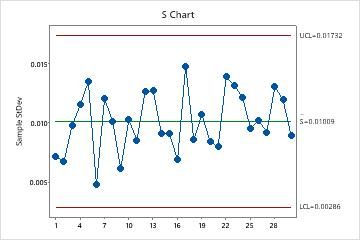In these results, the points vary randomly around the center line and are within the control limits. No trends or patterns are present. The variation of the process is stable enough to perform capability analysis..

## Moving range (MR) chart

The MR chart plots the moving ranges. The center line is the average of all moving ranges. The control limits, which are set at a distance of 3 standard deviations above and below the center line, show the amount of variation that is expected in the moving ranges.

Minitab displays an MR chart If your subgroup size is 1.

### Interpretation

Use an MR chart to monitor the variation (moving range) of your process and to determine whether it is stable enough to perform capability analysis. The MR chart indicates whether the variation from observation to observation is in control.

Points located beyond the red control limits indicate that the process may not be stable and that the results of a capability analysis may not be reliable. You should identify the cause of out-of-control points and eliminate special-cause variation before you analyze process capability.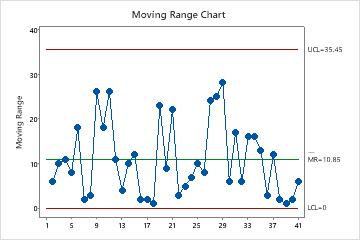In these results, the points vary randomly around the center line and are within the control limits. No trends or patterns are present. The variation of the process is stable enough to perform capability analysis.

## Last 25 Subgroups plot

The Last 25 Subgroups plot shows the data points for each of the last 25 subgroups and displays a line for the overall process mean.

### Interpretation

Use the Last 25 Subgroups plot to evaluate whether the distribution of the observations within the subgroups meets the requirements for your capability analysis.

The data should be randomly and symmetrically distributed about the process mean. Check the plot for evidence of the following:
• A subgroup that contains outliers
• Distribution changes across subgroups

If the plot shows evidence of problems or changes across subgroups, the capability results may not be valid. Investigate your process to determine why the data values are not distributed randomly across the subgroups.

###### Note

If your data has subgroup size of 1, Minitab displays a Last 25 Observations plot, which is interpreted similarly.

## Capability Histogram

The capability histogram shows the distribution of your sample data. Each bar on the histogram represents the frequency of data within an interval. The solid red curve represents the nonnormal distribution model that was selected for the analysis.

### Interpretation

Use the capability histogram to visualize your sample data in relation to the distribution fit and the specification limits.

To visually assess the distribution fit, compare the bars in the histogram with the curved fit line. The shape of the data in the histogram should approximately match the curve. To ascertain whether the data follow the distribution, use the results in the probability plot.

Visually examine the data in the histogram in relation to the lower and upper specification limits. Ideally, the spread of the data is narrower than the specification spread, and all the data are inside the specification limits. Data that are outside the specification limits represent nonconforming items.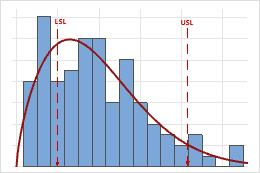In these results, the process spread is larger than the specification spread, which suggests poor capability. Although much of the data are within the specification limits, there are many nonconforming items below the lower specification limit (LSL) and above the upper specification limit (USL).
###### Note

To determine the number of nonconforming items in your process use the overall PPM result.

## Probability Plot

A probably plot displays each data point versus the percentage of values in the sample that are less than or equal to that data point. A probability plot includes the following components:
Middle line
The expected percentile from the distribution based on maximum likelihood parameter estimates.
Confidence bound lines
The left curved line indicates the lower bounds of the confidence intervals for the percentiles. The right curved line indicates the upper bounds of the confidence intervals for the percentiles.
Anderson-Darling test statistic and p-value
The results of a test to determine whether your data follow the distribution.

### Interpretation

Use the probability plot to assess the fit of the nonnormal distribution used for the analysis.

If the distribution is a good fit for the data, the points should form an approximately straight line. Departures from this straight line indicate that the fit is unacceptable. If the p-value is greater than 0.05, you can assume that the data follow the nonnormal distribution used in the analysis.

If the p-value is less than 0.05, your data do not follow the selected distribution and the capability analysis results may not be accurate. Use Individual Distribution Identification to determine which nonnormal distribution or data transformation is more effective for your data.

## Capability Plot

The capability plot is located at the lower right corner of the Nonnormal Capability Sixpack Report.